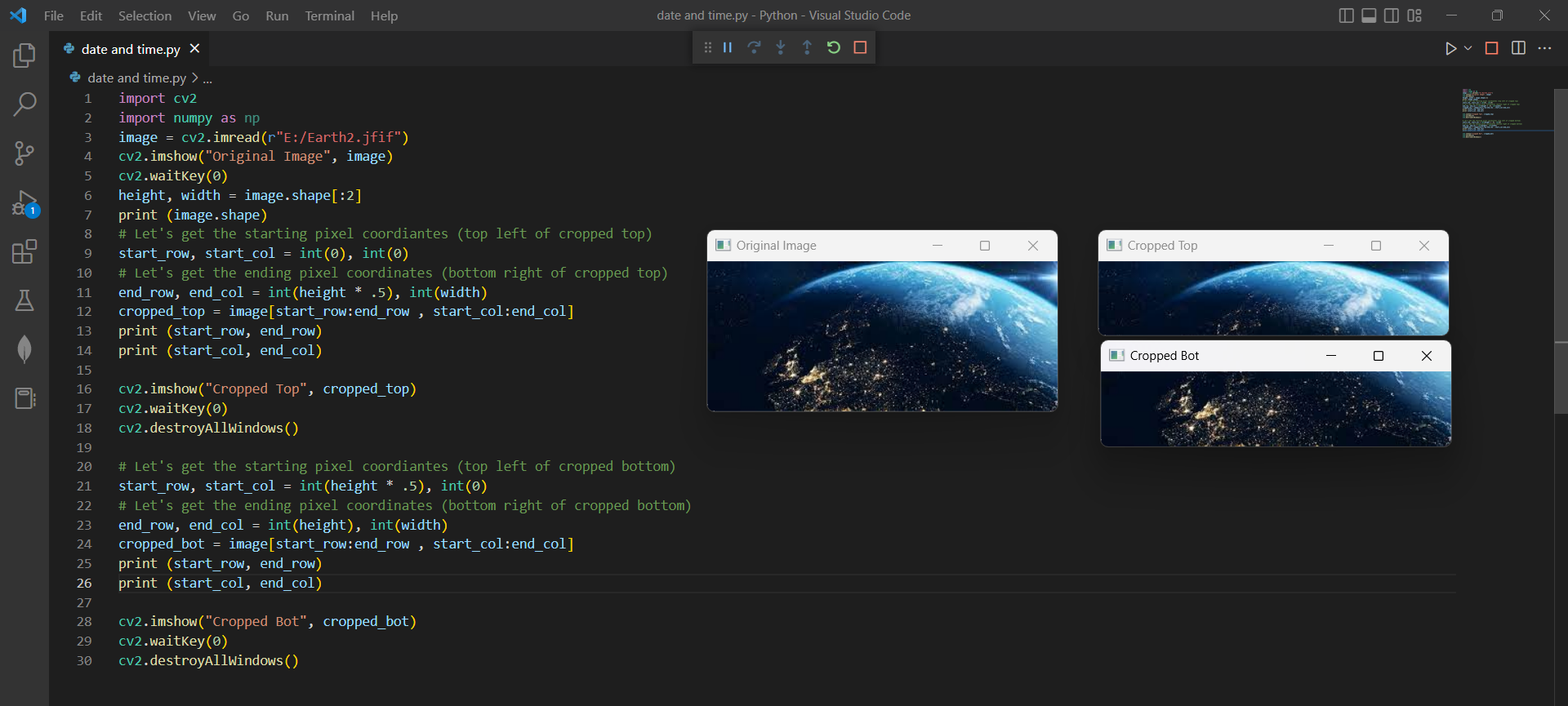# Divide Image into Two equal Parts using Open Cvby vigneshchennai74Updated: Feb 6, 2023Solution Kit

OpenCV is a library of programming functions mainly aimed at real-time computer vision. It is written in C, C++, and Python, and runs on Windows, Linux, Android, and macOS.OpenCV is widely used in the field of computer vision for tasks such as object recognition, face detection, and image and video analysis. It has a large community of developers and users and is continuously updated and improved.

OpenCV provides a large collection of algorithms and functions for image and video processing, including:

• Image processing operations like filtering, morphological transformations, thresholding, etc.
• Object detection and recognition, including face detection and recognition, object tracking, etc.
• Image and video analysis, including edge detection, feature extraction, and optical flow.
• Camera calibration and 3D reconstruction.
• Machine learning algorithms, including support for deep learning frameworks like TensorFlow and Caffe.

You can divide an image into two equal parts vertically or horizontally using OpenCV by simply slicing the image array. Here's an example of how you could divide an image into two equal parts horizontally in Python using OpenCV:

This code splits the image into two equal parts, horizontally. It first retrieves the shape of the image and calculates the height and width of the image. It then calculates the starting and ending row and column pixel coordinates for the top and bottom halves of the image. The image is then sliced and each half is stored in the cropped_top and cropped_bot variables. Finally, each of the two cropped images is displayed using the OpenCV function cv2.imshow() and is shown until a key is pressed using the cv2.waitKey(0) function

Here is an example of how you can Divide the image into two equal parts using OpenCVPreview of the output that you will get on running this code from your IDE

CODE

In this solution we use the Imread function of the OpenCV.

1. Copy the code using the "Copy" button above, and paste it in a Python file in your IDE.
2. Modify the name, location of the image to display in the code.
3. Run the file to divide the image to Top and Bottom

I hope you found this useful. I have added the link to dependent libraries, version information in the following sections.

i found this code snippet by searching for "divide image into tow equal parts python opencv" in kandi. You can try any such use case!

### Environment Tested

I tested this solution in the following versions. Be mindful of changes when working with other versions

1. The solution is created and executed in python version 3.7.15 .
2. The solution is tested on OpenCV 4.6.0
3. The solution is tested on numpy 1.21.6

Using this solution, we are able to divide an image using the OpenCV library in Python with simple steps. This process also facilities an easy-to-use, hassle-free method to create a hands-on working version of code which would help us divide an image in Python

### Dependent Library

opencvby opencv

C++69194Version:4.7.0Open Source Computer Vision Library

Support
Quality
Security
Reuse

opencvby opencv

C++69194Version:4.7.0License: Permissive (Apache-2.0)

Open Source Computer Vision Library
Support
Quality
Security
Reuse

numpyby numpy

Python23663Version:v1.25.0rc1The fundamental package for scientific computing with Python.

Support
Quality
Security
Reuse

numpyby numpy

Python23663Version:v1.25.0rc1License: Permissive (BSD-3-Clause)

The fundamental package for scientific computing with Python.
Support
Quality
Security